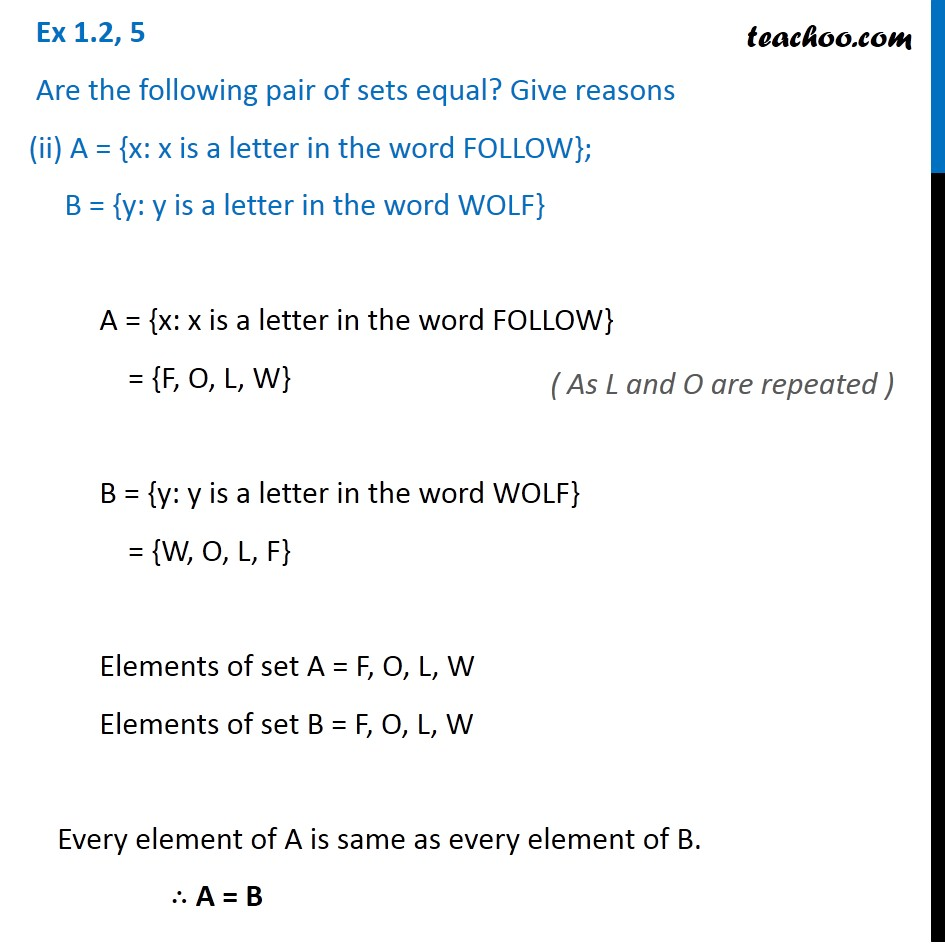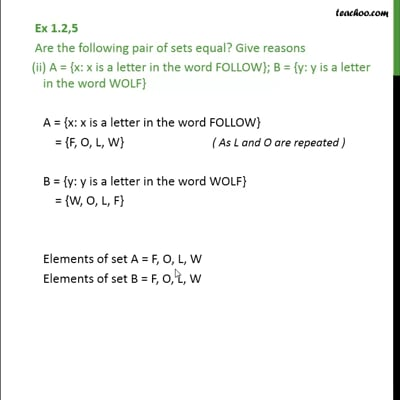Ex 1.2

Chapter 1 Class 11 Sets
Serial order wiseThis video is only available for Teachoo black users

Maths Crash Course - Live lectures + all videos + Real time Doubt solving!

### Transcript

Ex 1.2, 5 Are the following pair of sets equal? Give reasons (ii) A = {x: x is a letter in the word FOLLOW}; B = {y: y is a letter in the word WOLF} A = {x: x is a letter in the word FOLLOW} = {F, O, L, W} B = {y: y is a letter in the word WOLF} = {W, O, L, F} Elements of set A = F, O, L, W Elements of set B = F, O, L, W Every element of A is same as every element of B. ∴ A = B